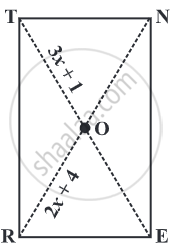Advertisement Remove all ads

# RENT is a rectangle. Its diagonals meet at O. Find x, if OR = 2x + 4 and OT = 3x + 1. - Mathematics

Sum
RENT is a rectangle. Its diagonals meet at O. Find x, if OR = 2x + 4 and OT = 3x + 1.Advertisement Remove all ads

#### Solution

bar"OT"  "is half of the diagonal"  bar"TE".

bar"OR"  "is half of the diagonal"  bar"RN".

by the property of Diagonals of a Rectangle Are of Equal Length.

So, their halves are also equal.
Therefore,
3x + 1 = 2x + 4
3x - 2x = 4 - 1
x = 3.
Is there an error in this question or solution?
Advertisement Remove all ads

#### Video TutorialsVIEW ALL 

Advertisement Remove all ads
Share
Notifications

View all notifications

Forgot password?
Course